MLA
APA
EndNote（.enw）

# 如何用方程式寫春聯

2015/02/22 | | 標籤：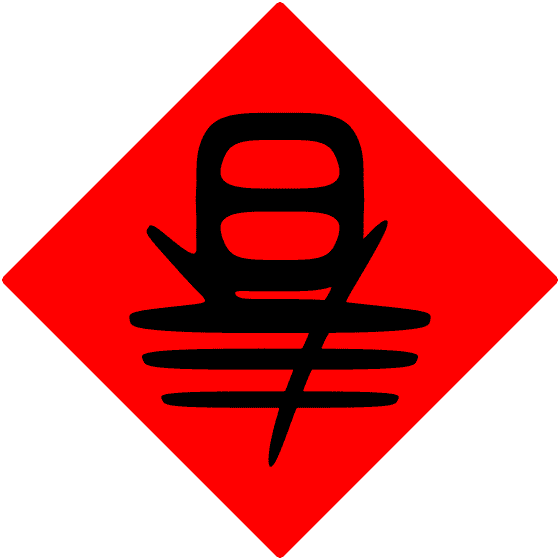文/ Simbol 1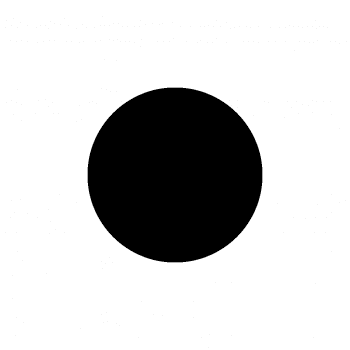x^4+y^4<25 倒是剛剛好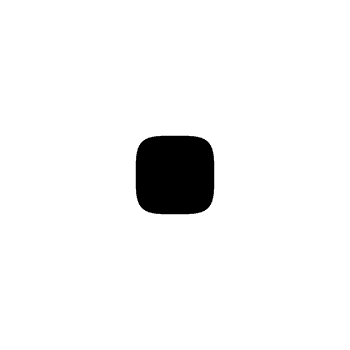x^4+y^4+100/(x^4+y^4)<25 的圖案長這樣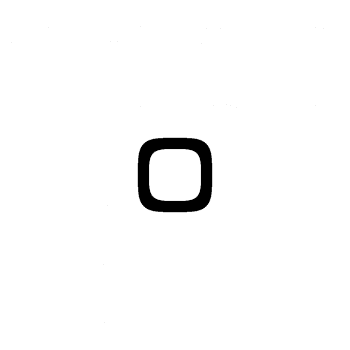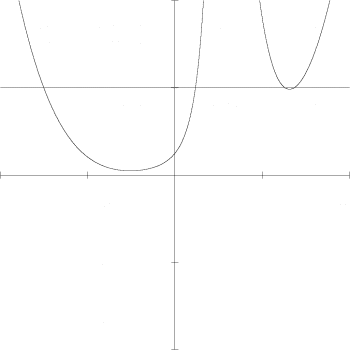(-5y+2(x/4)^4+100/((x/4)^4+(2(x/4)-2)^4+(2(x/4)-1)^2))(-5y+25)=0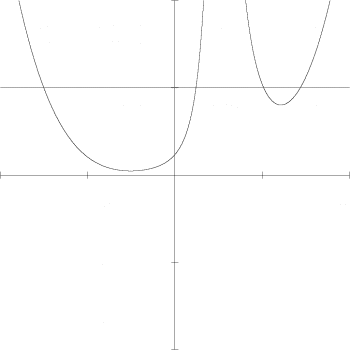x^4+y^4+100/(x^4+(2y-2)^4+(2y-1)^2)+100/(x^4+(2y+2)^4+(2y+1)^2)<25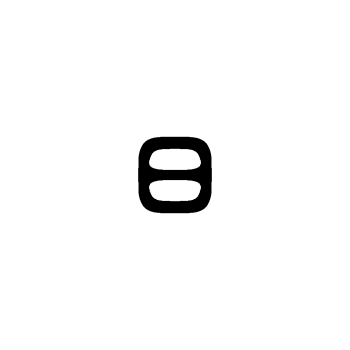（知道這三條分別對應到哪一項嗎？）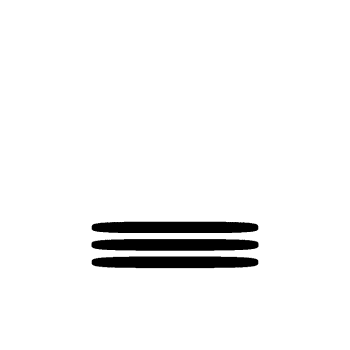x^4+y^4-1/((y+3)^4+(x/15)^4)-1/((y+4)^4+(x/15)^4)-1/((y+5)^4+(x/15)^4)<25x^4+y^4+100/(x^4+(2y-2)^4+(2y-1)^2)+100/(x^4+(2y+2)^4+(2y+1)^2)-1/((y+3)^4+(x/15)^4)-1/((y+4)^4+(x/15)^4)-1/((y+5)^4+(x/15)^4)<25(-50x+y^4+100/((2y-2)^4+(2y-1)^2)+100/((2y+2)^4+(2y+1)^2)-1/((y+3)^4)-1/((y+4)^4)-1/((y+5)^4))(-50x+25)=0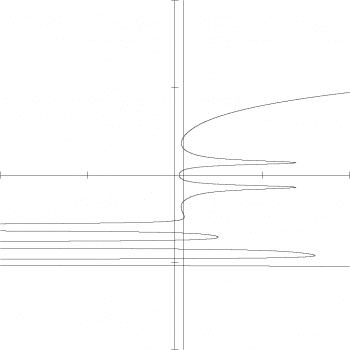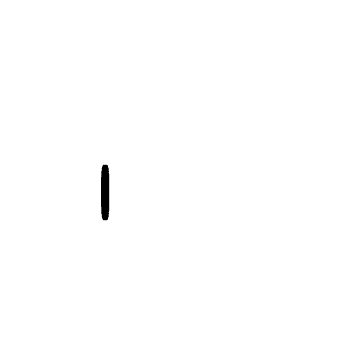-1/(4(x+y+4)^4+((x-y+1)/5)^4)<-100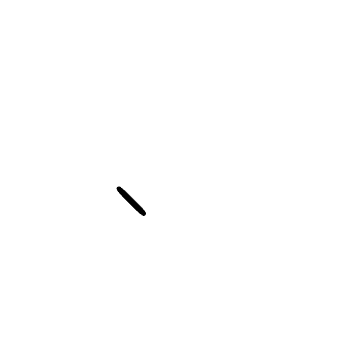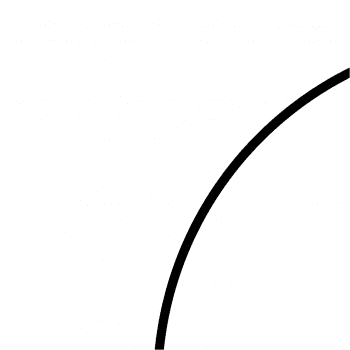((x-4)^2+(y+5)^2-13)((x-19)^2+(y+12)^2-400)=0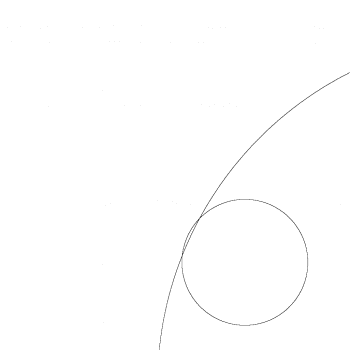((x-4)^2+(y+5)^2-13)^2+((x-19)^2+(y+12)^2-400)^2<100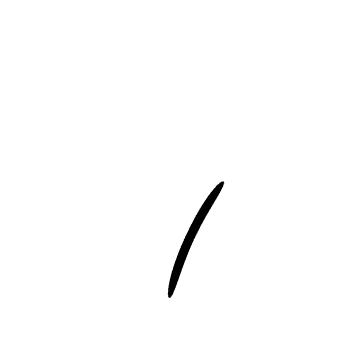-100/(((x-4)^2+(y+5)^2-13)^2+((x-19)^2+(y+12)^2-400)^2)<-1
（需要看看它的樣子嗎？我猜是不用。）

-100^16/(((x-4)^2+(y+5)^2-13)^2+((x-19)^2+(y+12)^2-400)^2)^16<-100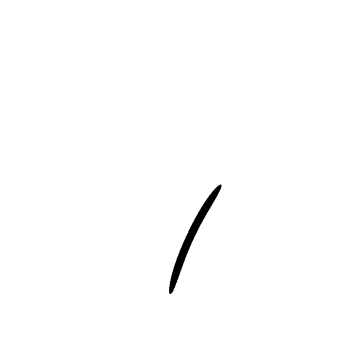（這個圖形和前一個一樣嗎？）
（如果不，那它為什麼和前一個那麼像？）

x^4+y^4+100/(x^4+(2y-2)^4+(2y-1)^2)+100/(x^4+(2y+2)^4+(2y+1)^2)-1/((y+3)^4+(x/15)^4)-1/((y+4)^4+(x/15)^4)-1/((y+5)^4+(x/15)^4)-1/(4(x+y+4)^4+((x-y+1)/5)^4)-100^16/(((x-4)^2+(y+5)^2-13)^2+((x-19)^2+(y+12)^2-400)^2)^16<25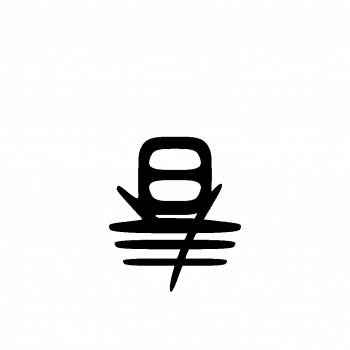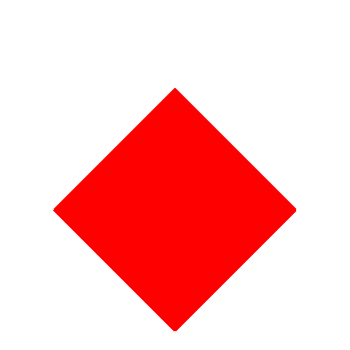x^4+y^4+100/(x^4+(2y-2)^4+(2y-1)^2)+100/(x^4+(2y+2)^4+(2y+1)^2)-1/((y+3)^4+(x/15)^4)-1/((y+4)^4+(x/15)^4)-1/((y+5)^4+(x/15)^4)-1/(4(x+y+4)^4+((x-y+1)/5)^4)-100^16/(((x-4)^2+(y+5)^2-13)^2+((x-19)^2+(y+12)^2-400)^2)^16-25

＿＿＿＿＿＿＿＿＿＿＿＿＿＿＿＿＿＿＿

## 關於作者